# AlgebraPage 1

#### WATCH ALL SLIDES

Slide 1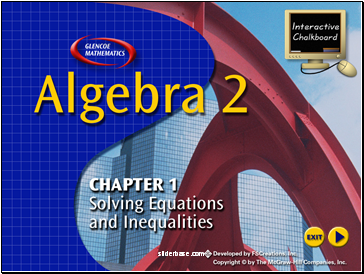Splash Screen

Slide 2Contents

Lesson 1-1 Expressions and Formulas

Lesson 1-2 Properties of Real Numbers

Lesson 1-3 Solving Equations

Lesson 1-4 Solving Absolute Value Equations

Lesson 1-5 Solving Inequalities

Lesson 1-6 Solving Compound and Absolute Value Inequalities

Slide 31 Contents

Example 1 Simplify an Expression

Example 2 Evaluate an Expression

Example 3 Expression Containing a Fraction Bar

Example 4 Use a Formula

Slide 4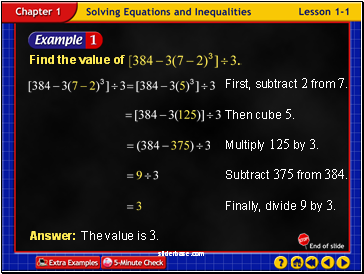Example 1-1a

Slide 5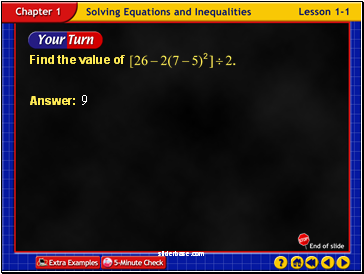Example 1-1b

Slide 6Example 1-2a

Slide 7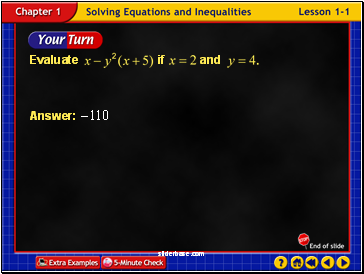Example 1-2b

Slide 8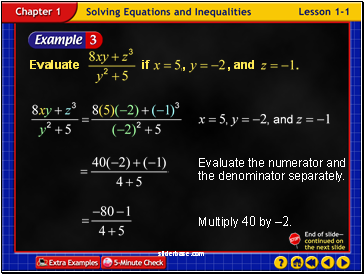Example 1-3a

Slide 9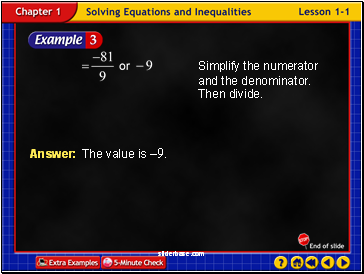Example 1-3b

Slide 10Example 1-3c

Slide 11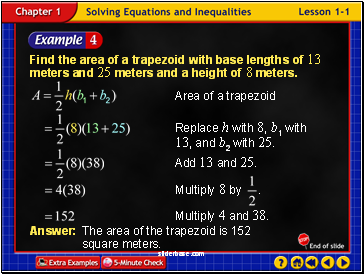Example 1-4a

Find the area of a trapezoid with base lengths of 13 meters and 25 meters and a height of 8 meters.

Answer: The area of the trapezoid is 152 square meters.

Slide 12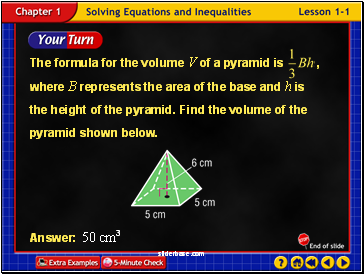Example 1-4b

Slide 13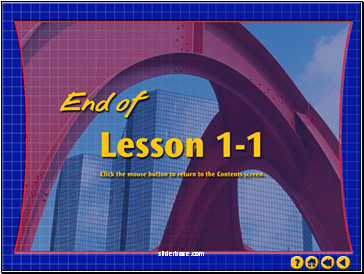End of Lesson 1

Slide 14Lesson 2 Contents

Example 1 Classify Numbers

Example 2 Identify Properties of Real Numbers

Example 3 Additive and Multiplicative Inverses

Example 4 Use the Distributive Property to Solve a Problem

Example 5 Simplify an Expression

Slide 15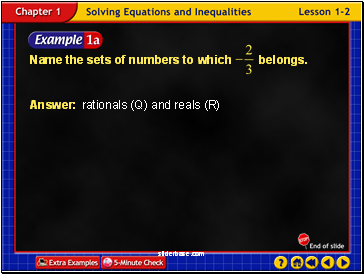Example 2-1a

Answer: rationals (Q) and reals (R)

Slide 16Example 2-1b

Answer: rationals (Q) and reals (R)

The bar over the 9 indicates that those digits repeat forever.

Go to page:
1  2  3  4  5  6  7  8